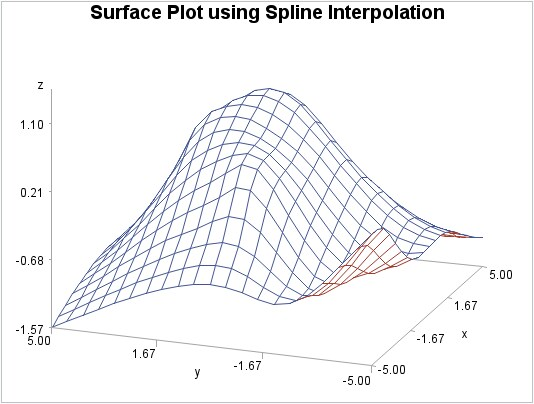Example 2: Spline and Smoothing Interpolations

 Features: GRID statement options SMOOTH= and SPLINE Data set: NUMS Sample library member: GTGSISS
This example extends Using the Default Interpolation Method to specify the SPLINE option on the GRID statement. The output data set, when used in PROC G3D, generates a smoother surface plot.
Surface Plot using Spline Interpolation (gtgsiss)The following plot extends Using the Default Interpolation Method to specify the SPLINE option, and the SMOOTH= option on the GRID statement. The SMOOTH= option is set to .05 for additional smoothing. The output data set, when used in PROC G3D, generates a smoother surface plot.
Surface Plot using Spline Interpolation and .05 Smoothing (gtgsiss)Program

goptions reset=all  border;
title "Surface Plot using Spline
Interpolation";
proc g3grid data=nums out=spline;
grid y*x=z / spline
axis1=-5 to 5 by .5
axis2=-5 to 5 by .5;
run;
proc g3d data=spline;
plot y*x=z ;
run;
quit;
title "Surface Plot using Spline Interpolation and
.05 Smoothing";
proc g3grid data=nums out=smoothed;
grid y*x=z / spline
smooth=.05
axis1=-5 to 5 by .5
axis2=-5 to 5 by .5;
run;
quit;
proc g3d data=smoothed;
plot y*x=z;
run;
quit;

Program Description

Set the graphics environment.
goptions reset=all  border;
Define the title for the plot.
title "Surface Plot using Spline
Interpolation";
Process points with PROC G3GRID. The SPLINE option specifies the bivariate spline method for the data set interpolation.
proc g3grid data=nums out=spline;
grid y*x=z / spline
axis1=-5 to 5 by .5
axis2=-5 to 5 by .5;
run;
Generate the surface plot.
proc g3d data=spline;
plot y*x=z ;
run;
quit;
Define the title for the plot.
title "Surface Plot using Spline Interpolation and
.05 Smoothing";
Process the data with PROC G3GRID.The SMOOTH=.05 option specifies the smoothing parameter to use during spline interpolation.
proc g3grid data=nums out=smoothed;
grid y*x=z / spline
smooth=.05
axis1=-5 to 5 by .5
axis2=-5 to 5 by .5;
run;
quit;
Generate the surface plot.
proc g3d data=smoothed;
plot y*x=z;
run;
quit;